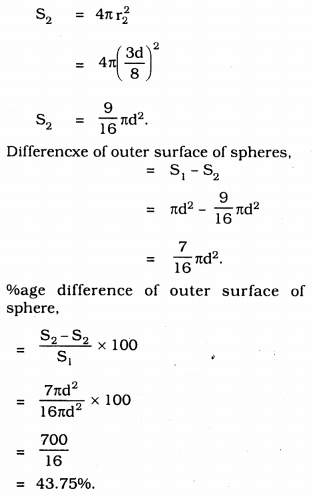# KSEEB Solutions for Class 9 Maths Chapter 13 Surface Areas and Volumes Ex 13.9

## Karnataka Board Class 9 Maths Chapter 13 Surface Areas and Volumes Ex 13.9

Question 1.
A wooden bookshelf has external dimensions as follows:
Height = 110 cm, Depth = 25 cm, Breadth = 85 cm.The thickness of the plank is 5 cm everywhere. The external faces are to be polished and the inner faces are to be painted. If the rate of polishing is 20 paise per cm2 and the rate of painting is 10 paise per cm2, find the total expenses required for polishing and painting the surface of the bookshelf.
Solution:
Outer length, l = 25 cm.
outer breadth, b = 85 cm.
outer height, h = 110 cm.
Outer surface area = lh + 2lb + 2bh = lh + 2(lb + bh)
= 85 × 110 + 2(85 × 25 + 25 × 110)
= 9350 + 9750
= 19100 cm2.
Area of front face,
= [85 × 110 – 75 × 100 + 2(75 × 5)]
= (9350 – 7500 + 2(375)]
= 9350 – 7500 + 750
= 11000 – 7500
= 3500 cm2.
Area to be polished,
= 19100 + 3500
= 22600 cm2.
Cost of polishing 1 cm3 is Rs. 0.20.
Cost of polishing 22600 cm3 … ? …
= 22600 × 0.20 = Rs. 4520.
Length of horizontal shelf, l = 75 cm.
height, h = 30 cm.
Area of horizontal shelf
= 2(l + h)b + lh
= [2(75 + 30) × 20 + 75 × 30]
= (4200 + 2250] cm2.
= 6450 cm2.
∴ Area of painting 3 horizontal rows
= 3 × 6450
= 19350 cm2.
Cost of painting for 1 cm3 is Rs. 0.10.
∴ Cost of painting 19350 cm3 … ?
= 19350 × 0.10 = Rs. 1935.
∴ Total cost of polish and painting
= Rs. 4520 + Rs. 1935
= Rs. 6455.

Question 2.
The front compound wall of a house is decorated by wooden spheres of diameter 21 cm, placed on small supports as shown in Fig.Eight such spheres are used for this purpose and are to be painted silver. Each support is a cylinder of radius 1.5 cm and height 7 cm and is to be painted black. Find the cost of paint required if silver paint costs 25 paise per cm2 and black paint costs 5 paise per cm2.
Solution:
Diameter of a wooden frame, d = 21 cm.
Radius r = $$\frac{21}{2}$$
Outer surface area of wooden spheres,
A = 4πr2∴ A = 1386cm2
Radius of cylinder, r1 = 1.5 cm
height, h = 7 cm
The curved surface area of cylinder support,
A = 2πrhArea of silver painted
= [8 × (1386 – 7.07)] cm2
= 8 × 1378.93
= 11031.44 cm2
Cost of silver paint = 11031.44 × 0.25
= Rs. 2757.86
Area of black paint = (8 × 66) cm2
= 528 cm2
Cost of black paint = 528 × 0.05
= Rs. 26.40
Total cost of silver and black paint.
= Rs. 2757.76 + Rs. 26.40
= Rs. 2784.26.

Question 3.
The diameter of a sphere is decreased by 25%. By what percent does its curved surface area decrease?
Solution:
Let the diametrer of sphere be ‘d’
Radius of sphere, r1 = $$\frac{\mathrm{d}}{2}$$
Radius of outer sphere, r2 = $$\frac{\mathrm{d}}{2}\left(1-\frac{25}{100}\right)$$
∴ r2 = $$\frac{3}{8}$$d
Outer Area of Sphere, S1 = 4πr12
= 4π$$\left(\frac{\mathrm{d}}{2}\right)^{2}$$
S1 = πd2
The diameter of sphere is decreased by 25%. Then its outer surface area,##### KSEEB Solutions for Class 9 Maths

error: Content is protected !!